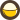點餐

1. 沖繩阿古豚肉丼
\$108
2. 德島県香魚爐端燒定食
\$138
3. 北海道帶子香草醬扁意粉
\$108
4. 明太子鮮蝦扁意粉
\$108
5. 蕃茄海鮮扁意粉
\$108
6. 煙肉蘑菇蛋汁扁意粉
\$108
7. 冬蔭功鮮蝦扁意粉
\$108

1. 燒物 - 北海道元貝
\$88
2. 燒物 - 手打鮮墨魚棒
\$68
3. 燒物 - 蟹味噌甲羅燒
\$88
4. 燒物 - 喜之次一夜干
\$548
5. 燒物 - 酒場一夜干
\$198
6. 燒物 - 德島県香魚
\$78
7. 燒物 - 松葉蟹腳
\$328
8. 燒物 - 七味魔鬼魚翅干
\$88
9. 燒物 - 明太子
\$88
10. 燒物 - 蠔
\$58
11. 燒物 - 虎蝦
\$78

1. 一蔵串燒盛合 雞翼 X 1、牛舌 X 1、免治雞肉棒 X 1、日本甜薯 X 1、冬菇 X 1
\$188
2. 一蔵野菜盛合 青椒 X 1、大蔥 X 1、秋葵 X 1、日本甜薯 X 1、冬菇 X 1
\$128
1. 串燒 - 厚燒牛舌
\$78
2. 串燒 - 免治雞肉棒
\$48
3. 串燒 - 雞翼
\$38
4. 串燒 - 青椒仔
\$32
5. 串燒 - 秋葵
\$32
6. 串燒 - 椎茸
\$32
7. 串燒 - 日本甜薯
\$32
8. 串燒 - 大蔥
\$32
9. 串燒 - 牛小排
\$68
10. 串燒 - 雞軟骨
\$32
11. 串燒 - 雞腎
\$32
12. 串燒 - 雞心
\$32
13. 串燒 - 豚肉生菜
\$48
14. 串燒 - 豚肉露荀
\$48
15. 串燒 - 洋蔥
\$32

1. 醬油大腸
\$62
2. 豚舌慢煮
\$62
3. 明太子玉子燒
\$62
4. 粟米天婦羅
\$62
5. 海苔竹輪唐揚
\$52
6. 海苔芝士脆
\$52
7. 煎餃子
\$62
8. 沖繩豚肉丼
\$118

1. 鮟鱇魚肝
\$78
2. 瑩光魷魚沖漬
\$58
3. 酒漬雞肝
\$48
4. 牛蒡漬
\$38
5. 蒜頭味噌漬
\$38
6. 柚子秋葵漬
\$38
7. 山葵八爪魚
\$38

1. 凍柚子蜜
\$28
2. 熱柚子蜜
\$28
3. 凍柑桔蜜
\$28
4. 熱柑桔蜜
\$28
5. 鮮檸梳打
\$28
6. 七喜
\$18
7. 可樂
\$18
\$0